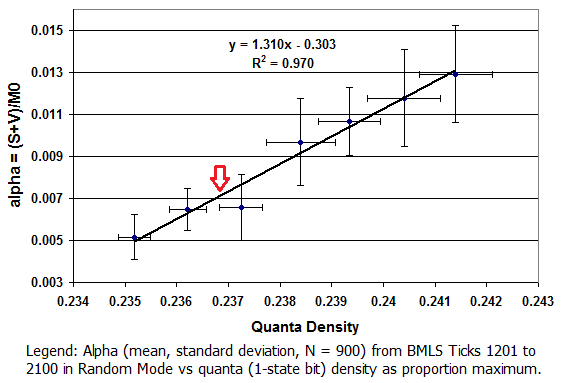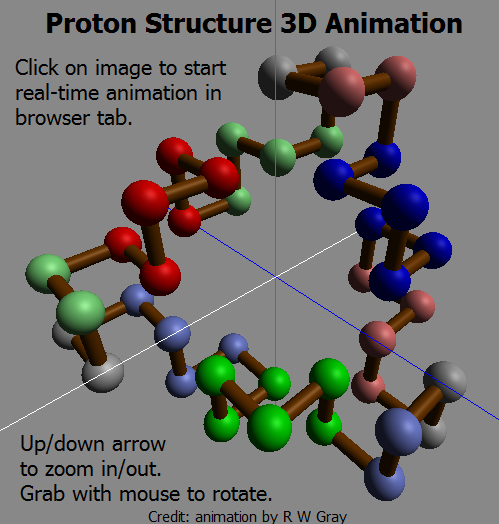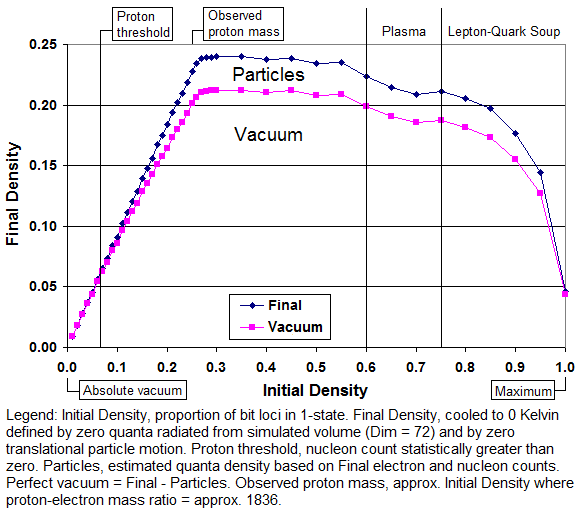## Wednesday, November 4, 2020

### Physicists Had A Chance And They Blew It

Editorial
The first-ever derivation of so-called fundamental constants from first principles of quantum theory  was a historic event. The first of over 90 papers on binary mechanics appeared in 2010 in JBinMech. But the first paper deriving Planck's constant h was not published until 2015. So physicists around the world had some five years to win the century-long physics grand championship race. A major missed opportunity. They had a chance and they blew it. Instead, Binary Mechanics Lab (BML) crossed the finish line and won the greatest race in physics in over 100 years .

Table 1: Unexplained Measurements Wrongly Called "Fundamental Constants"

## Sunday, November 1, 2020

### Binary Mechanics Postulates

Abstract and Introduction
In "Binary mechanics", written in 1994 and published in 2010 , the eight-component wave function of a pair of relativisitic Dirac spinor equations of opposite handedness was parsed to define the spot cube model of space. With quantization of energy, space and time, dubbed full quantization, the spot cube provided a new system state representation, called the bit function, at a quantized time. With full quantization, infinitesimal increments in the Dirac equation pair were no longer applicable. Hence, time-development of the system state was defined in four bit operations. The postulates of binary mechanics define primary constants from full quantization and the mathematical definitions of the bit function and bit operations .

Fig. 1: Spot Cube Model of Space

## Thursday, October 22, 2020

### Fundamental Physical Constants Doctrine

Abstract and Introduction
The fundamental physical constants doctrine hides the failure of popular physical theories including legacy quantum mechanics and both special and general relativity. The so-called "fundamental constants" are in fact the greatest body of unexplained data in physics. The doctrine acts to obscure the now obvious fact that these unexplained observations comprise basic unsolved mysteries in physics. Instead of addressing these basic questions, the doctrine teaches that these observations are essentially a sort of "no-go zone" for theoretical physicists. First, these observations are typically confined to a short Appendix A in physics books, wrongly labelled "fundamental constants" (Fig. 1). Second, "natural units" in basic equations help hide the fact that the measured values of these "constants" remains unexplained although these values are used as "input parameters". Third, accepted interrelationships or dependencies among many of these constants indicate mathematically that they contain redundant information and could not be "fundamental". Finally, binary mechanics is thus far the only comprehensive physical theory to derive the values of the so-called fundamental constants from first principles .

Fig. 1: The Greatest Body Of Unexplained Data In Physics

## Monday, June 29, 2020

### Motion Law: Gravitation Edition

Abstract and Introduction
As a consequence of binary mechanics (BM) fundamentals , a motion law states that objects tend to move in the direction of higher vacuum energy density . As background, topics discussed include particles as compositions of multiple quanta, the mechanism of particle movement as a flux of individual quanta , the most likely motion direction and the equivalence of the gravitational field within a solid object and a quanta density gradient in its perfect vacuum component . Predictions from this model have been confirmed by experimental results of Alex L Dmitriev et al, reporting weight decease with a (1) heated brass rod, (2) heating a piezo ceramic pile, (3) laser injection in optical fibers and (4) in gyros proportional to spin frequency and with horizontal more than vertical spin axis. The role of temperature in gravity-like effects has now been studied in two broad categories: distant objects not in direct contact and the special case of a weighed object resting on a scale.

Fig. 1: Motion Law At Single Particle Level## Saturday, June 20, 2020

### Fine Structure Constant Derivation

Abstract and Introduction
Some consequences of defining the fine structure constant α as the probability of an electromagnetic interaction with a charged particle are explored using the Binary Mechanics Lab Simulator (BMLS) v2.8. An alpha α composite variable was introduced: (S + V) / M0, where S and V are scalar (electrostatic) and vector (magnetic) event counts respectively and M0 is the number of M-type quanta (1-state bits with charge attribute) prior to application of time-development bit operations and eligible to be "source quanta" in the S and V bit operations . In brief, this α definition is simply the observed probability that a M quanta is accelerated by an electrostatic (S) or magnetic (V) potential. The α variable was not constant, but varied as a function of quanta density in the simulated volume (Fig. 1), suggesting that α may have appeared to be constant if previous measurements were conducted at a quanta density of approximately 0.237 of maximum possible density. Proton-electron mass ratio was also found to occur at about the same quanta density suggesting that this density range may approximate laboratory conditions close to "standard temperature and pressure".

Fig. 1: Fine Structure Constant α vs Quanta Density## Friday, May 22, 2020

### Proton Structure 3D Animation

Abstract and Introduction
The proton (hadron) bit cycle was rendered in a 3D animation illustrating features of the binary mechanics (BM) model of space   and proton structure, discovered in 2011   and used in the first-ever derivation of Planck's constant from first principles of quantum theory in 2018 .

Fig. 1: Proton Bit Cycle Viewed Along Spin AxisLegend: Spheres, 42 bit loci in matter d quark (dark red, green, blue), anti-matter d quark (light red, green, blue) and positron (grey) spot types. Brown bars, route of quanta in the proton cycle. Axis lines, X (blue), Y (pink) and Z (white) intersect at center of "home" spot cube, where the spin axis is approximately perpendicular to the page plane in this perspective.

## Monday, May 4, 2020

### Inertial Propulsion Mechanism

Abstract and Introduction
Inertial Propulsion may be described as conversion of angular momentum to linear momentum thus violating Newton’s mechanics in which these momentum types are separately conserved . For example, Eric Laithwaite  and others have demonstrated translation motion of a gyro only when spinning called “precession”, as well as apparent levitation. Unlike Newton’s mechanics, the time-development laws of binary mechanics (BM)   do not specifically require separate conservation of angular and linear momentum. However, energy (1-state bits called quanta) is conserved in BM. In fact, the BM time-development laws produce quanta motion alternating routinely between circular motion and translation and are the mechanism of inertial propulsion.

Fig. 1: Circular and Translation Quanta Motion in Electron CycleLegend: 3 spot units in electron spot (yellow). Centers of M and L bit loci size L cubes (white circles/arrows respectively) are equidistant from, and orthogonal to, the spin axis (grey circle) which is a spot cube solid diagonal (orthogonal to the spin and page planes).

## Monday, February 24, 2020

### Light Speed Derivation

Abstract
Physics literature presents equations in which a measured physical constant is expressed as one or more other measured physical constants. These expressions (1) show dependencies among so-called "fundamental" constants which are in fact unexplained observations and (2) are not derivations from first principles. That is, a true derivation from first principles cannot use any unexplained data as one or more "input" parameters. Adding to previous reports  , a procedure to derive light speed with unidirectional measurements is described based only on the first principles of binary mechanics including the time-development laws  and a physical interpretation of binary mechanical space .

Introduction
With first principles describing electron geometry, zero electron electric dipole moment was derived in 2011  and confirmed by two different labs . With the discovery of the proton (hadron) bit cycle in 2011 , the non-spherical proton shape was described, confirmed by proton scattering data .

Using the classical definition of total angular momentum, intrinsic electron spin and hence, Planck's constant, were derived in 2015 . In 2018, Planck's constant and both electron and proton intrinsic spin where derived using a different method by summation of the angular momentum of each quanta motion in the electron and proton bit cycles . Fractional and elementary charge derivation was based on analysis of the time-development scalar (electrostatic) bit operation  and paved the way to derive intrinsic electron magnetic moment based solely on first principles, the elementary charge derivation and the classical definition of magnetic dipole moment .

These first-ever derivations of previously unexplained constants required full quantization of energy, space and time, namely the units of measurement in physics (Fig. 1). A primary constant value for each unit of measurement could be assigned that was consistent with the full set of derivations -- mass M as energy expressed in kg, length L in meters and time T in seconds . These three values may complete the list of primary constants, if fine-structure constant α in Fig. 1 can also be successfully derived from first principles [Keene, in preparation].

## Wednesday, January 1, 2020

### JBinMech 2020

Eight papers and research reports in 2020 further cemented the clear lead of JBinMech as the premier journal for milestone advances in particle and theoretical physics.

Light speed has been an unexplained observation wrongly characterized as a "fundamental physical constant" . Hence, "Light speed derivation"  and previous reports deriving light speed for the first time from first principles, using three different measurement methods, may represent a remarkable, even stunning, development in this story that merits careful examination. "Binary mechanics postulates"  summarized these first principles based on quantization of space, time and energy, dubbed full quantization, in 17 simple equations without any reference to, or use of, unexplained measurements (c, h, e, me, etc), traditionally called fundamental constants, as input parameters.

"Fine structure constant derivation"  provided a credible, possible definition of the α value based only on binary mechanics postulates.

Binary mechanics postulates do not require that linear and angular momentum are separately conserved, as Newton's work is commonly presented. While macroscopic observations generally support the idea of separate conservation of linear and angular momentum, the inertial propulsion phenomenon in gyroscope behavior raised questions about this popular assumption. "Inertial propulsion mechanism"  documented how quanta (1-state bit loci) may routinely exchange linear and angular momentum components at the quanta microscopic level.

Boeing uses "inertial propulsion gyroscopes" to "levitate" low altitude satellites which would otherwise fall to earth prematurely, but can't explain it. "Motion law: gravitation edition"  used the motion law of binary mechanics to describe the fundamental mechanism for weight reduction produced by gyroscopes with horizontal rotors, citing experimental results.

"Proton structure 3D animation"  presented a 3D animation of proton structure and discussed key features determining observed proton behavior and why QCD (quantum chromodynamics) became obsolete due to use of legitimate, but inapplicable, math tools.

Finally, an editorial, "Physicists had a chance and they blew it" , described how the physics community missed a major opportunity. Instead, BML crossed the finish line and won the greatest race in physics in over 100 years. However, the good news is the abundance of additional opportunities -- more constants to derive from first principles, more detailed examination and replication of many pilot studies in JBinMech, more fundamental questions to address with further research, problems in all specialties in physics. Many new races to win.

References
 Keene, J. J. "Fundamental physical constants doctrine" JBinMech October, 2020.
 Keene, J. J. "Light speed derivation" JBinMech February, 2020.
 Keene, J. J. "Binary mechanics postulates" JBinMech November, 2020.
 Keene, J. J. "Fine structure constant derivation" JBinMech June, 2020.
 Keene, J. J. "Inertial propulsion mechanism" JBinMech May, 2020.
 Keene, J. J. "Motion law: gravitation edition" JBinMech June, 2020.
 Keene, J. J. "Proton structure 3D animation" JBinMech May, 2020.
 Keene, J. J. "Physicists had a chance and they blew it" JBinMech November, 2020.

## Monday, December 9, 2019

### Vacuum Composition

Abstract and Introduction
Assertions that perfect vacuum and almost all of the volume of a single atom are "empty space" are questionable. In a replication of a previous simulation experiment  with additional analysis, perfect vacuum was defined as total energy density minus electron and nucleon particle density. Examining the entire range of non-zero energy quanta (1-state bit) densities, only about 12 percent or less of the quanta were associated with particles, indicating that perfect vacuum was composed of about 88 percent or more of quanta in the final state after cooling (Figs. 1 and 2). Threshold energy density for baryogenesis (nucleon formation) was 0.07 of maximum. In higher energy density initial states in the plasma and lepton-quark soup ranges, "explosive" centrifugal momentum leaves much lower particle and vacuum energy densities after cooling, which may be relevant to expanding universe questions.

Fig. 1: Vacuum Composition After Cooling to Zero Kelvin (Final Density)## Thursday, August 1, 2019

### Recent BML Videos

Brief History of Quantum Theory

## Wednesday, March 27, 2019

### Electron Energy Homeostasis

Abstract and Introduction
Quanta absorption by electron spots represents "quanta capture" by the electron bit cycle as described previously  . In electron spots with E = 1 or E = 2, where E is number quanta (0 to 6), quanta absorption and emission events exhibit homeostatic properties which act to regulate or limit spot energy content. These effects are consistent with accepted models that absorption yields an excited electron state and emission represents return to a ground state. The present analysis aims to clarify the physical mechanisms in these processes.

Fig. 1: Quanta Capture by Electron Spot